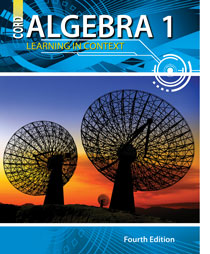# Algebra 1 - 4th Edition

## Chapter 2: Solving Equations

Some links are repeated for use with more than one lesson.

### These sites apply to the entire chapter.

http://www.sosmath.com/algebra/solve/solve0/solve0.html

http://www.bbc.co.uk/education/mathsfile/shockwave/games/equationmatch.html
This site contains a game to practice solving equations by matching. This website requires Shockwave Player to run some of its features.

### 2.1 Properties of Multiplication and Division

http://www.aaamath.com/pro74b-propertiesmult.html
This site explains the properties of multiplication and has a game to test knowledge of them.

http://www.sparknotes.com/math/prealgebra/operations/section3.rhtml

### 2.2 Solving Proportions and Percent Equations

http://www.mathgoodies.com/lessons/percent/proportions.html

### 2.3 Properties of Addition and Subtraction

http://www.aaastudy.com/pro74ax2.htm
This site explains the properties of addition and has a game to test knowledge of them.

http://science.jrank.org/pages/6576/Subtraction-Properties.html

### 2.4 Multistep Equations

http://www.algebralab.org/lessons/lesson.aspx?file=algebra_onevariablemultistep.xml
This article explains how to solve multistep equations as well as having vocabulary terms.

http://www.purplemath.com/modules/solvelin2.htm
This site explains how to solve multistep equations by working out examples and showing the steps.

http://www.algebra.com/algebra/homework/Linear-equations/linear-equation.solver
Online solver, showing steps to solve any one-variable linear equation.

### 2.5 Equations with Variables on Both Sides

http://www.algebralab.org/studyaids/studyaid.aspx?file=Algebra1_3-3.xml
This page explains in detail how to solve equations with variables on both sides and has examples with answers.

http://www.mathvids.com/lesson/mathhelp/204-solving-with-variables-on-both-sides
This site contains a video tutorial with examples of solving equations with variables on both sides.

### 2.6 Solving Absolute Value Equations

http://www.coolmath.com/algebra/Algebra1/05AbsValueEq/02_equations.htm
This page contains notes on how to solve absolute value equations and has several examples of fully solved problems.

http://www.purplemath.com/modules/solveabs.htm